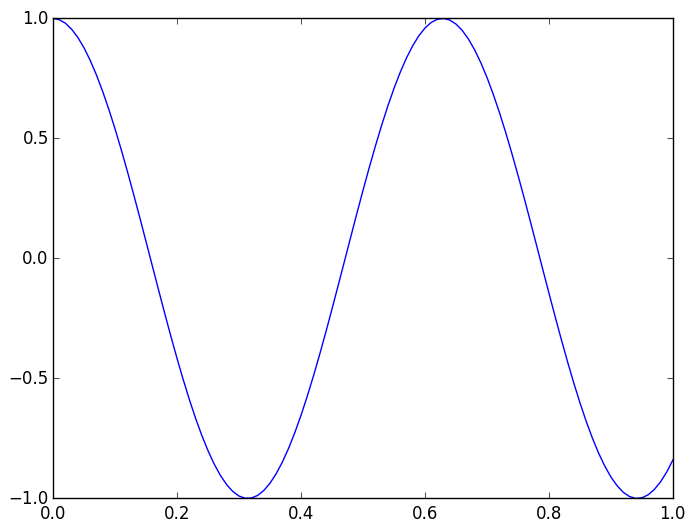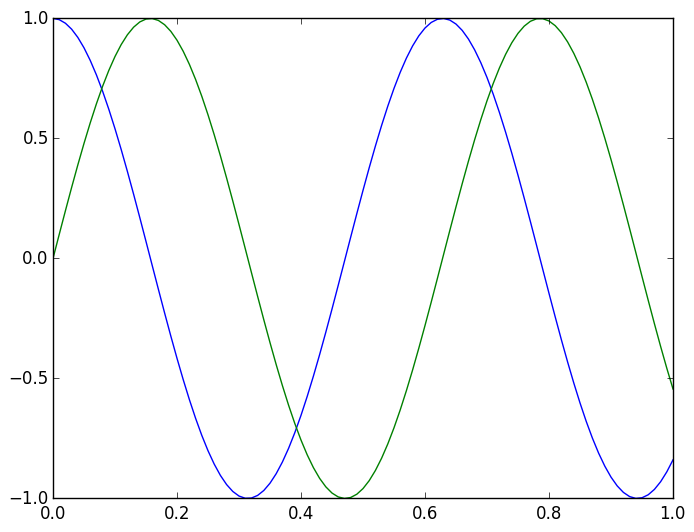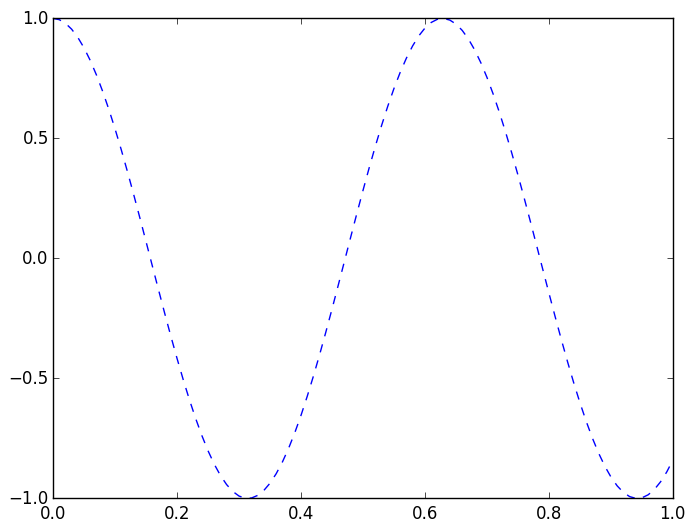# Lab 1¶

In this tutorial, we will familiarize ourselves with using Julia, both on the command line and in Jupyter. Before we begin, please run the following command in the terminal:

cp -r /nb/m3976/.local ~/

## Julia from the command line¶

If you open a terminal, you can run julia by typing

    julia

This opens what's called a REPL prompt: a Read-Evaluate-Print loop, where Julia waits for you to type a command and hit enter.

Try typing some commands at the Julia REPL prompt, such as:

1+1

In :
bits(52)

Out:
"0000000000000000000000000000000000000000000000000000000000110100"

The lab matchines default to 32 bit integers, so your output will be different. Can you predict what the output of the following will be before hitting return?

In [ ]:
bits(11)

In [ ]:
bits(-11)


multiple lines can be input on the same line using ;. For example, the following assigns the value of 2 to x and then prints out x+2:

In :
x=2; x+2

Out:
4

; also has the feature of surpressing the output. What do you think the output of the following is:

In :
x=2; x+2;


## Creating a Julia script¶

Julia can also run a script that is in a file. At a Julia prompt type

quit()


to go back to the unix terminal. We can create a simple script. Type

emacs myfirstscript.jl &

to create a Julia script file named myfirstscript.jl and edit it. In the emacs window, type

println("hello world")


Save the file by typing Ctrl-X Ctrl-S.

We can now run the script by evaluating:

julia myfirstscript.jl

from the terminal.

## Creating a Julia file with a function defined on it¶

We can also create files that define Julia functions, that can be loaded from the REPL. To see this, type

emacs myfirstfunction.jl &

in the terminal and type the following to create a function called sq, just like in lecture:

function sq(x)
x^2
end


Save (Ctrl-X Ctrl-S) the file.

Nothing happens if we type

julia myfirstfunction.jl

in the terminal. Why is this?

julia

to open up the Julia REPL. Typing

include("myfirstfunction.jl")


in Julia is the same as evaluating the contents of the file on the command line prompt. We can now use sq, by typing

sq(5)  # returns 25


Excercise 1 Edit myfirstfunction.jl to add a function sqbits(x), that returns the bits of sq(x). Load myfirstfunction.jl in a new REPL, and check that sqbits(5) returns "0000000000000000000000000000000000000000000000000000000000011001"

Excercise 2 Create a new function that sets the 10th bit of an Int32 to 1, and returns an Int32, assuming that the input is a positive integer. Two strings can be concatenated with *:

"hi"*"bye" # returns the string "hibye"


The string consisting of the first nine characters can be found using str[1:9] where str is any string:

str="hibye0123445556"
str[1:9]  # returns "hibye0123"


The string consisting of the 11th through last character can be found using str[11:end]:

str="hibye0123445556"
str[11:end]  # returns "45556"


The function

parse(Int32,str,2)

converts from a string in Base-2 to an Int32.

Excercise 3 (advanced) Modify the previous function to also work with negative numbers. Recall that

reinterpret(Int32,x)

will reinterpret the bits of number x which is an unsigned integer UInt32 as if it were a signed integer Int32.

## Jupyter notebook¶

The interface we will use mostly is the Jupyter notebook. Julia support is provided by the Julia package IJulia.
To open up a Jupyter notebook, type the following:

using IJulia
notebook()


This will open up a new firefox window showing the contents of the current directory. If you click on New in the top right and select Julia 0.4.3, a new window will open that let's you type in Julia commands.

Try typing in some of the commands at the start of the notebook. You can create new boxes by clicking on the + in the toolbar, or hitting Ctrl-m b. Cells can be deleted by clicking the scissors in the tool bar, or Ctrl-m x.

### Copying Jupyter notebooks¶

This tutorial guide is itself a Jupyter notebook. To download, click the image that looks like a down arrow. A cryptic bunch of text should come up. Hit Ctrl-S and save the file in your home directory as "01.ipynb"

You can now open this tutorial from the Jupyter notebook website. Click on jupyter in the top left from the Jupyter window to return to the directory window, and click on "01.ipynb". Try evaluating some of the boxes above. Scroll down to here in the file.

# Plotting¶

A nice thing about Jupyter is that it supports plotting. Let's do an easy example:

In :
using PyPlot  # Loads the PyPlot Package

In :
x=0.:.01:1.    # Represents a range of x values, with step size 0.01
y=cos(10*x)    # returns [cos(10*0.),cos(10*0.01),cos(10*0.02),cos(10*0.03),…,cos(10*1.)]
plot(x,y)      # plots cos(10*x) between 0 and 1;Multiple plots can be combined. Note that the variables x and y are saved.

In :
plot(x,cos(10x))
plot(x,sin(10x));We can plot points by specifying linestyle='.':

In :
plot(x,cos(10x); linestyle="--");Excercise 4 Plot the digits of a random integer in base-10. Hint: the function rand(Int) creates a random integer, and digits(x) returns a vector of the digits.

Excercise 5 Plot the digits of 5 random integers in base-10, using a for-loop. Hint: remember the syntax

for k=1:5
# do stuff
end